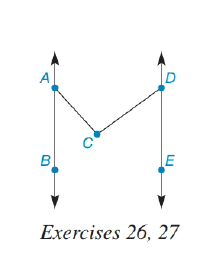Chapter 2.1, Problem 25E### Elementary Geometry for College St...

6th Edition
Daniel C. Alexander + 1 other
ISBN: 9781285195698

#### Solutions

Chapter
Section### Elementary Geometry for College St...

6th Edition
Daniel C. Alexander + 1 other
ISBN: 9781285195698
Textbook Problem
3 views

# Given: A B ↔ ∥ D E ↔ m ∠ B A C + m ∠ C D E = 93 ° Find: m ∠ A C D (See “Hint” in Exercise 26.)To determine

To find:

The value of mACD.

Explanation

Given:

The given figure is,

Figure (1)

And,

ABDE, mBAC+mCDE=93°.

Concept:

If two lines are parallel lines are cut by a transversal then the angles of the alternate sides of transverse lines are equal.

Construction:

Draw a straight line passing through point C in such a way that it is parallel to lines AB and DE and name it as FG.

Figure (2)

Calculation:

Consider the figure (2),

mACF+mDCF=mACD …… (1)

Alternate angles are congruent

### Still sussing out bartleby?

Check out a sample textbook solution.

See a sample solution

#### The Solution to Your Study Problems

Bartleby provides explanations to thousands of textbook problems written by our experts, many with advanced degrees!

Get Started

#### Find the derivative of the function. U(y)=(y4+1y2+1)5

Single Variable Calculus: Early Transcendentals, Volume I